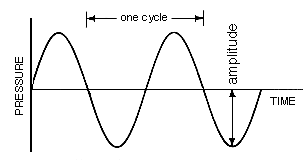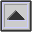AMPLITUDE

The maximum deviation from the average or equilibrium value of any repeatedly changing quantity, such as the position of a vibrating object, pressure, velocity, voltage, current and many others. The amplitude of a SOUND WAVE is the maximum amount by which the instantaneous SOUND PRESSURE differs from the AMBIENT pressure. See diagram under SIMPLE HARMONIC MOTION.Two CYCLEs of a sine wave showing the amplitude of the pressure variation.

The variation of (maximum) amplitude over time is called the ENVELOPE of the sound.

home